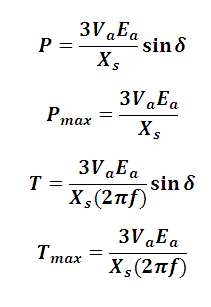# Power and torque in Synchronous Motor Calculator

This Calctown Calculator calculates the power delivered by a synchronous motor.

V
V
deg
ohm
Hz

#### Result

W
N-m
W
N-mwhere

E = Excitation voltage

V = terminal or supply voltage

X = reactance

δ = power angle

P = power

T = torque